# Texas Go Math Kindergarten Unit 1 Assessment Answer Key

Refer to our Texas Go Math Kindergarten Answer Key Pdf to score good marks in the exams. Test yourself by practicing the problems from Texas Go Math Kindergarten Unit 1 Assessment Answer Key.

## Texas Go Math Kindergarten Unit 1 Assessment Answer Key

Vocabulary

DIRECTIONS: 1. Draw lines to match the counters ¡n the ten frames to the number word. 2. Count and tell how many. Write the number. (TEKS K.2.B) 3. Start with 15. Count forward. Write numbers in order. (TEKS K.2.B)

Question 1.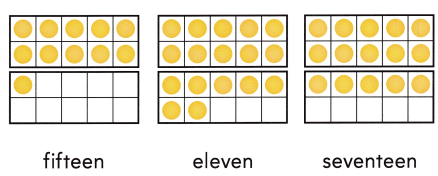Explanation:
First frame number of counters = 10 + 1 = 11 or Eleven.
Second frame number of counters = 10 + 7 = 17 or Seventeen.
Third frame number of counters = 10 + 5 = 15 or Fifteen.

Concepts and Skills
Question 2.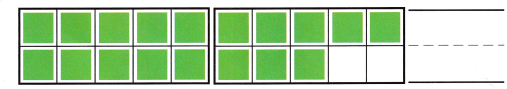Explanation:
Number of cubes = 10 + 8 = 18 or Eighteen.

Question 3.Explanation:
Numbers after 15 = 15, 16, 17, 18, 19, 20.

DIRECTIONS: 4. Draw more flowers to show 14 flowers. (TEKS K.2.G) 5-6. Mark under the number that shows how many. (TEKS K.2.B) 7. Mark under the set that models the number at the beginning of the row. (TEKS k.2.G) 8. Mark under the numbers that show them in order. (TEKS K.2.A)

Question 4.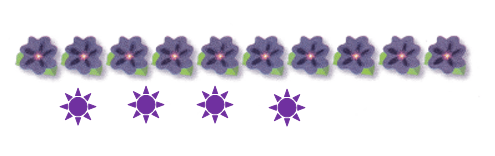Explanation:
Number of flowers = 10 + 4 = 14 or Fourteen.

Question 5.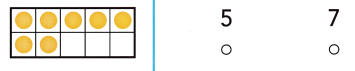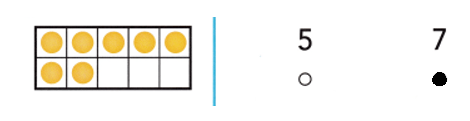Explanation:
Number of counters = 5 + 2 = 7 or Seven.

Question 6.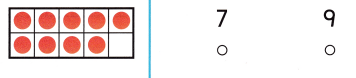Explanation:
Number of counters = 5 + 4 = 9 or Nine.

Question 7.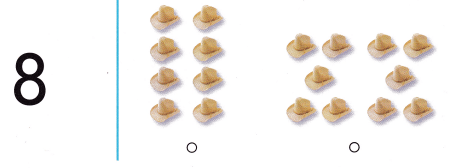Explanation:
Number of things = 8 or Eight.

Question 8.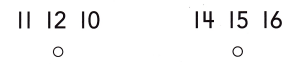Explanation:
Numbers in order =  14, 15, 16 not 11, 12, 10.

DIRECTIONS: 9. Mark under the set that shows the number at the beginning of the row. (TEKS K.2.B) 10. Mark under the number that shows how many. (TEKS K.2.G) 11. Mark beside the number that is one more. (TEKS K.2.F) 12. Mark beside the number that is one less. (TEKS K.2.F)

Question 9.Explanation:
Number of counters = 14 or Fourteen.

Question 10.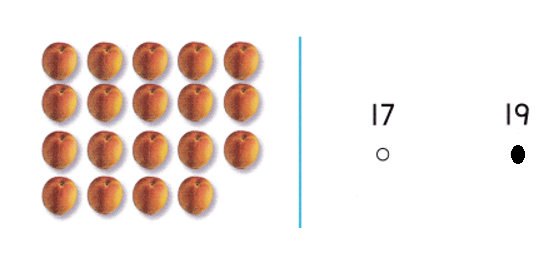Explanation:
Number of apples = 10 + 9 = 19 or Nineteen.

Question 11.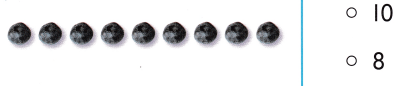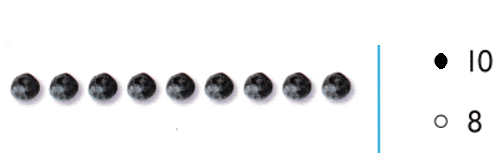Explanation:
One more than 9 = 9 + 1 = 10 or Ten.

Question 12.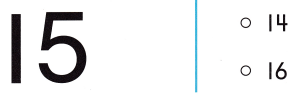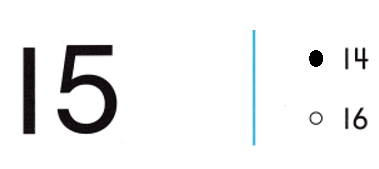Explanation:
One less than 15 = 15 – 1 = 14 or Fourteen.